[中文] Search Internet Search HelloDBA
Oracle Technology Site. email: fuyuncat@gmail.com  MSN: fuyuncat@hotmail.com# CBO Statistics data gathering and internal arithmetic analysis -- Sampling

[中文]

Author:  fuyuncat

Source:  www.HelloDBA.com

Date:  2010-01-20 07:34:44

Share to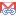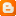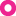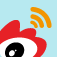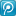## Sampling

When analyzing objects, to reduce workload, we may analyze with sampling data to estimate the statistics data. This may reduce the accuracy, but will save much of resource and reduce loading in server, which are important for analyzing the huge tables.

When set sampling size, we have 3 choices.

•   Set a specified sample size, from 0.000001 to 100;
•   set as DBMS_STATS.AUTO_SAMPLE_SIZE or 0, which will let oracle choose the sample size automaticly;
•   no setting, null or 100, means will no sampling.

If set as AUTO, Oracle will have a quick estimating table rows base on data blocks, and then set a initial sampling percent dependent on the row number. To get the row number, oracle will scan the sample data iterativly with adjusting the sampling percent. Here is the pseudocode.

SQL代码
1. set initial sample pct (NPCT) by Number of Blocks (NBS) # NPCT = LEAST(100, GREATEST(0.000001, (100 * 100 / NBS)))
2. loop {
3.   if NPCT > 25 then
4.     set NPCT = 100
5.   if NPCT >= 100 then
6.     set the query SQL as "select count(*) from table"
7.   else
8.     set the query SQL as "select count(*) from table sample(NPCT)"
9.   execute SQL and get the sample records number (SN)
10.   if SN > 5000 or NPCT >= 100 then
11.     exit loop
12.   else
13.     if SN >= 441 then
14.       NPCT = GREATEST((NPCT*7500/SN), (NPCT*10))
15.     else
16.       NPCT = NPCT*100;
17. }
18. Get the estimated records number # N = SN*100/NPCT

We can find the loop from the trace.

SQL代码
1. ...
2. quick_estimate_rowcnt: 4359 rows seen at  14.7058823529 percent (aprx. 100 blocks)
3. quick_estimate_rowcnt: 47582 rows seen at 100 percent (aprx. 680 blocks)
4. ...

With the estimated row number, oracle will set the initial percent by below formula. We can see, in AUTO sampling, the table with less 5500 records will almost not be sampled.
PCT = 5500*100/N

For the previous example, it's initial percent is,
PCT = 5500*100/47582 = 11.558992896473456349039552772057

However, with the initial percent (including the manually specified percent), the analyzed statistics data may not accurate enough to be adopted. In such case, oracle will adjust the percent and re-analyze those data. We can find the re-analyzing iterations from the trace.

SQL代码
1. Iteration 1, percentage 11.55899289647345634903955277205666008154
2.  NNV  NDV  AVG  MMX  HST  EP   RP   NNNP IND  CNDV HSTN HSTR  COLNAME
3.   Y    Y    Y    Y    Y    Y    Y    Y    Y              Y    SYS_NC00015\$
4.        Y    Y    Y    Y    Y    Y    Y    Y    Y         Y    OWNER
5.        Y    Y    Y    Y    Y    Y    Y    Y    Y         Y    OBJECT_NAME
6.   Y    Y    Y    Y    Y    Y    Y                        Y    SUBOBJECT_NAME
7.        Y    Y    Y    Y    Y    Y    Y    Y              Y    OBJECT_ID
8.   Y    Y    Y    Y    Y    Y    Y                        Y    DATA_OBJECT_ID
9.   Y    Y    Y    Y    Y    Y    Y                        Y    OBJECT_TYPE
10.        Y         Y    Y    Y    Y         Y              Y    CREATED
11.        Y         Y    Y    Y    Y         Y              Y    LAST_DDL_TIME
12.   Y    Y    Y    Y    Y    Y    Y                        Y    TIMESTAMP
13.   Y    Y    Y    Y    Y    Y    Y         Y              Y    STATUS
14.   Y    Y    Y    Y    Y    Y    Y                        Y    TEMPORARY
15.   Y    Y    Y    Y    Y    Y    Y                        Y    GENERATED
16.   Y    Y    Y    Y    Y    Y    Y                        Y    SECONDARY
17. ...
18. Iteration 2, percentage 100
19.  NNV  NDV  AVG  MMX  HST  EP   RP   NNNP IND  CNDV HSTN HSTR  COLNAME
20.   Y    Y         Y    Y    Y    Y    Y    Y              Y    SYS_NC00015\$
21.                            Y    Y    Y    Y    Y         Y    OWNER
22.        Y         Y    Y    Y    Y    Y    Y    Y         Y    OBJECT_NAME
23.                            Y    Y                        Y    SUBOBJECT_NAME
24.        Y         Y    Y    Y    Y    Y    Y              Y    OBJECT_ID
25.   Y    Y    Y    Y    Y    Y    Y                        Y    DATA_OBJECT_ID
26.                            Y    Y                        Y    OBJECT_TYPE
27.                            Y    Y         Y              Y    CREATED
28.                            Y    Y         Y              Y    LAST_DDL_TIME
29.                            Y    Y                        Y    TIMESTAMP
30.                            Y    Y         Y              Y    STATUS
31.                            Y    Y                        Y    TEMPORARY
32.                            Y    Y                        Y    GENERATED
33.                            Y    Y                        Y    SECONDARY
34. ...

It will trace the gathering flag bits at the begining of each iteration. Oracle will use those bits to decide which statistics data should be analyzed. By comparing the bits of adjacent iterations, we will acknowledge which which statistics data will be re-analyzed.

Such as the sample size (SSIZE), sample number of not null values (SNNV) and calculated number of distinct values (NDV) are the data to be checked to decide will be percent be adjusted. Below are the pseudocode.

SQL代码
1. get initial sampling PCT, set as CURR_PCT
2. loop
3. {
4.   Get the basic statistics data
5.   set NEW_PCT = CURR_PCT * 10
6.   for all columns required histogram
7.   {
8.     if SNNV of the column < 441 then
9.     {
10.       Need re-analyzed
11.       NEW_PCT = GREATEST(PCT*441*1.25/GREATEST(SNNV, 1), NEW_PCT)
12.     }
13.     else
14.     {
15.       gather the column histogram with NEW_PCT
16.       if it's a Height Balanced Histogram and the NDV can not be scaled
17.         Need re-analyzed
18.     }
19.   }
20.
21.   If sample size of table < 441 then
22.   {
23.     Need re-analyzed
24.     NEW_PCT = GREATEST(PCT*441*1.25/GREATEST(SNNV, 1), NEW_PCT)
25.   }
26.   evaluate all columns' basic statistics data One By One
27.   {
28.     if column required average size or max/min size then
29.       if the original PCT is auto and column's SNNV < 919 then
30.       {
31.         Need re-analyzed
32.         NEW_PCT = GREATEST(PCT*441*1.25/GREATEST(SNNV, 1), NEW_PCT)
33.       }
34.
35.     if column required NDV then
36.       If NDV can not be scaled
37.         Need re-analyzed
38.   }
39.
40.   exit if is not auto sample
41.   if Need re-analyzed then
42.     if current sample size >= 441 and NEW_PCT > 25 and NEW_PCT < 50 and CURR_PCT <=5 then
43.       NEW_PCT = 25
44.     PREV_PCT = CURR_PCT
45.     CURR_PCT = NEW_PCT
46.   else
47.     exit
48. }

We can also find the statistics data must be re-analyzed from the trace.

SQL代码
1. ...
2. Scaling NDV of OBJECT_NAME
3.  Need larger sample: sndv = 5128, snnvdv = 5401, nnv = 46726, ndv = 24986
4. ...

There is the sample size in both of the dictionary table of table (tab\$) and column (col\$).

SQL代码
1. HELLODBA.COM>select o.name, t.samplesize
2.   2    from tab\$ t, obj\$ o, user\$ u
3.   3   where t.obj# = o.obj#
4.   4     and o.owner# = u.user#
5.   5     and u.name = 'DEMO'
6.   6     and o.name = 'T_TEST1';
7.
8. NAME                           SAMPLESIZE
9. ------------------------------ ----------
10. T_TEST1                             47582
11.
12. HELLODBA.COM>select u.name, o.name, c.name, h.sample_size
13.   2    from hist_head\$ h, sys.col\$ c, obj\$ o, user\$ u
14.   3   where h.obj# = c.obj#
15.   4     and h.intcol# = c.intcol#
16.   5     and h.obj# = o.obj#
17.   6     and o.owner# = u.user#
18.   7     and u.name = 'DEMO'
19.   8     and o.name = 'T_TEST1';
20.
21. NAME                           NAME                           NAME                           SAMPLE_SIZE
22. ------------------------------ ------------------------------ ------------------------------ -----------
23. DEMO                           T_TEST1                        SYS_NC00015\$                         47582
24. DEMO                           T_TEST1                        OWNER                                 5401
25. DEMO                           T_TEST1                        OBJECT_NAME                          47582
26. DEMO                           T_TEST1                        SUBOBJECT_NAME                        5401
27. DEMO                           T_TEST1                        OBJECT_ID                            47582
28. DEMO                           T_TEST1                        DATA_OBJECT_ID                        5618
29. DEMO                           T_TEST1                        OBJECT_TYPE                           5401
30. DEMO                           T_TEST1                        CREATED                               5401
31. DEMO                           T_TEST1                        LAST_DDL_TIME                         5401
32. DEMO                           T_TEST1                        TIMESTAMP                             5401
33. DEMO                           T_TEST1                        STATUS                                5401
34. DEMO                           T_TEST1                        TEMPORARY                             5401
35. DEMO                           T_TEST1                        GENERATED                             5401
36. DEMO                           T_TEST1                        SECONDARY                             5401
37.
38. 14 rows selected.

The sample size of the table is the sample size in the last iteration, while the sample size of columns are the size of the analyzed statistics data on the column, including the histogram.

--- Fuyuncat  ---